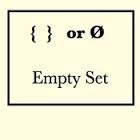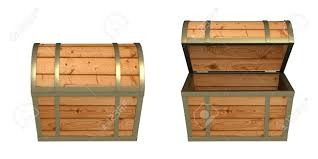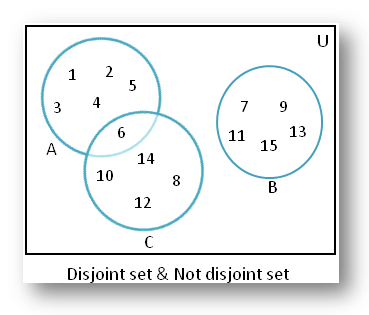×#### Thank you for registering.

One of our academic counsellors will contact you within 1 working day.

Click to Chat

1800-1023-196

+91-120-4616500

CART 0

• 0

MY CART (5)

Use Coupon: CART20 and get 20% off on all online Study Material

ITEM
DETAILS
MRP
DISCOUNT
FINAL PRICE
Total Price: Rs.

There are no items in this cart.
Continue Shopping```
The Empty Set

Table of Content

History of Empty set

Definition of Empty Set

Can you imagine the piano keys on a guitar?

Symbol or Notation of Empty Set

Cardinality of Empty Set

Example of Empty Set

Is the Empty Set, a subset of all sets?

Is the Empty Set, subset of itself?

What is the Power Set of an Empty Set?

Does Empty Set, contain 0 as an element?

Is the Null Set, an element of itself?

Is the Empty Set, both open and close?

Is the Empty Set, a vector space?

Representation of Empty Set with Venn diagram

History of Empty setThe Empty set was first derived by Leibniz while working on the initial conception of symbolic logic.

The Symbol of empty set (Ø) was introduced by the André Weil of the Bourbaki group in 1939.

Definition of Empty Set

The empty set is a unique set which have no element in it. Its size is zero i.e., the number of elements is 0.At some places, it is also called null set, but now null set is the concept of measure theory. So it is also called void set.

Well, the concept of Empty set is little weird. As we all know that, a set is a well-defined collection of distant objects, so how can it be possible a set having no element.

Can you imagine the piano keys on a guitar?No! It’s not possible. As this is a set with no required elements in it. So, it is the empty set.

Symbol or Notation of Empty Set

The empty set is denoted by { }, curly braces having no elements in it. It is like a box having nothing in it.​It is represented by symbol Ø (phi) (disambiguation) also.

Both { } and Ø have a same meaning.

Cardinality of Empty Set

The number of elements is the cardinality or size of a set. It is represented by |A| = x. The vertical bars here show the cardinality of a set ‘A’.

The cardinality of an empty set is zero because there is no element in the empty set.

Example

A= {a, e, i, o, u}

B= { }

Here,  |A| = 4

|B| = 0

Example of Empty Set

A set of even prime numbers more than 2.

A set of the months in a year starting with letter Z.

A set of all positive integers that is greater than their cubes.

Is the Empty Set, a subset of all sets?

To understand the answer of this question, first we need to understand the meaning of subset.

Subset – A set P is said to be a subset of a set Q if every element of P is also an element of Q. We use symbol “⊂” to represent a subset and symbol “∈ ” to represent ‘belong to’.In other words, P⊂Q if whenever x ∈P, then x ∈Q also. Generally, we use the symbol “⇒” which means implies. So, we can write the definition of subset as:

P⊂Q if x ∈P⇒ x ∈Q

We can read the above statement as “P is a subset of Q, if x is an element of P implies that x is also an element of Q”.

Example

Set A= {1, 2, 3, 4},

As all the elements belong to A have their own identity, so they can make their own sets. All the sets which have all the elements which belong to set A also are the subsets of A. The subsets of the above set are as follows:Here , we will write all the possible combinations. like first we will write sets with zero elements , then sets with 1 element, sets with 2 elements, sets with three elements and set with 4 elements.

It depends upon the number of elements of a set.It is not possible to list all the subsets everytime for large number of elements to see , how many subsets are there?

The number of subsets of a set with 1 elements is 2 only one empty set and other is the set itself.

The number of subsets of a set with 2 elements has 4 subsets.

The number of subsets of a set with 3 elements has 8 subsets.

The number of subsets of a set with 4 elements has 16 subsets.

Here we see that every time the munber of subsets are getting double.i.e., 2n.

So , we derived a formula to calculate the number of subsets having different number of elements.

The number of subsets of a set is calculated with the formula

If n (A) =z, then the number of subsets are 2z

In the above example,n (A) =4, so number of subsets are 24=16.

As in the above example of venn diagram,all the elements of a set P are present in set Q, so P is a subset of Q. And as empty set is a set which have no element in it, so it can be easily present in any set. So empty set is a subset of both P and Q.This shows that empty set is the subset of every set.

Is the Empty Set, subset of itself?

Every set is a subset of itself. As a set X is a subset of set Y,if all the elements of Y are there in X. So,it’s obvious that all the elements of X are there in X. So, X is also the subset of itself.

As in the above example, A = {1,2,3,4} and its one of the subset is also {1,2,3,4}.

So, this shows that every set is a subset of itself.

A ⊆ A and Ø ⊆ A

Here,we had learned that empty set is a subset of every set and every set is a subset of itself.

So, Empty set is also a subset of itself.

What is the Power Set of an Empty Set?

The set containing only the empty set is the power set of an empty set.

2Ø=Ø

As the power set is the set of all the subsets of any set. And empty set is the subset of itself so its power set will also be Ø, this only.

Does Empty Set contain 0 as an element?

To understand this, first we need to understand the difference between the empty set and the number zero.

If we say, A set of squares having five sides. But it’s not possible, so we will say that there are zero squares which have five sides, so it is an empty set.

But if we will write it as {0}, as the answer of this question then it will be wrong because it shows that it has an element but the empty set does not contain any element. So, it must be
{ }.Here, the number of element is zero but zero is not the element of this set.

Example

M= {0, 1, 2, 3, 4, 5, 6}

Here the number of elements is 7 and zero is one of the elements of Set M.If we write {0} as the subset of Set M, then it is not an empty set as it contains one element in it .But , as empty set is the subset of every set , so Ø is also the subset of M.So, both {0} and Ø are the subset of M but are not a same thing.

Zero is the number of element in empty set, not the element of empty set.

Is the Null Set, an element of itself?

The empty set is a set with no elements in it. So, there is zero number of elements in an empty set.

If we will say that null set is the element of itself, then we will write it as {Ø}. But it shows that it has an element in it which will be wrong in case of empty set.

Null set is the subset of itself but not the element of itself.

Is the Empty Set, both open and closed?It is very simple to understand the concept of open and closed empty set.

Here in the above picture, it shows one open and one closed box. So how does it matter rather it is open or closed if a box is empty. It will remain empty. If there is nothing in the box, it could be both open and closed.

In mathematical terms, a closed set is a set which has its limits and an open set is that which always have neighbor for its element.

And if a set has no boundaries it could be both open and closed.

There is no boundary for an empty set as there is no element in it.

So, the empty set is both open and closed set.

Is the Empty Set, a vector space?

A vector space is a set which is defined by two operations + (vector addition) and · (scalar multiplication).

If A is a vector space and B is a subset of A. Then B is a vector space with respect to the operations in A, and B is called a subspace of A.

As B is non-empty: The zero vector belongs to B.

But the empty set has no element in it, it is empty, hence it fails to have the zero vector as an element.

Since it does not contain zero vector, the empty set cannot be a vector space.

Representation of Empty Set with Venn diagram

Lets try to understand the concept of empty set through venn diagram.

Venn diagram is the graphical representation of sets.Here, A and B are two disjoint sets ,which are not intersecting each other, which means that there is no common element within them.So the number of same elements in those two sets will be 0(zero). Or you can say that the A intersection B is the empty set (no element).

As in the above picture,

A ∩ B= Ø

But, C and D are two joint sets which intersects each other. As in the above picture it represents the C ∩ D is the pink part, which shows that there are some common elements within C and D.

So, C ∩ D ≠ Ø i.e.,the intersection of C and D is not empty.There must be some element in it.

ExampleFor picture given in the right,

A = {1, 2, 3, 4, 5, 6}

B = {7, 9, 11, 13, 15}

C = {6, 8, 10, 12, 14}

Here,

A ∩ C = {6}, as 6 is the common element in A and C.

i.e.,  A ∩ C ≠ Ø

But,

A∩ B = Ø, as there is no common element in A and B.

B∩ C = this is also an empty set as both B and C also have no common element.

The Empty Set
```### Course Features

• 731 Video Lectures
• Revision Notes
• Previous Year Papers
• Mind Map
• Study Planner
• NCERT Solutions
• Discussion Forum
• Test paper with Video Solution